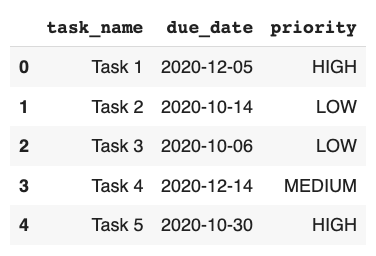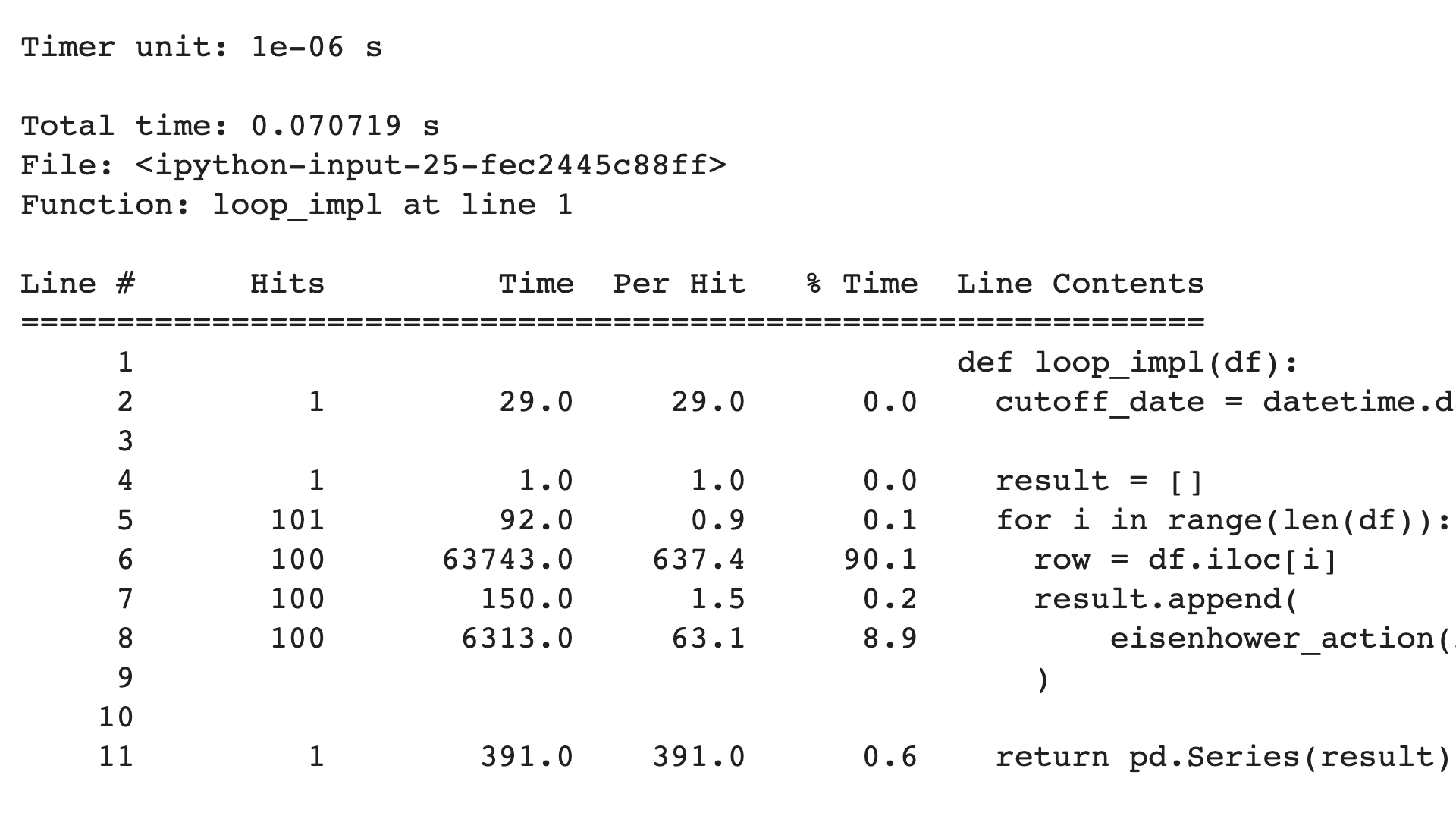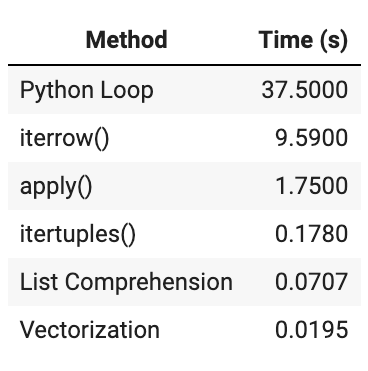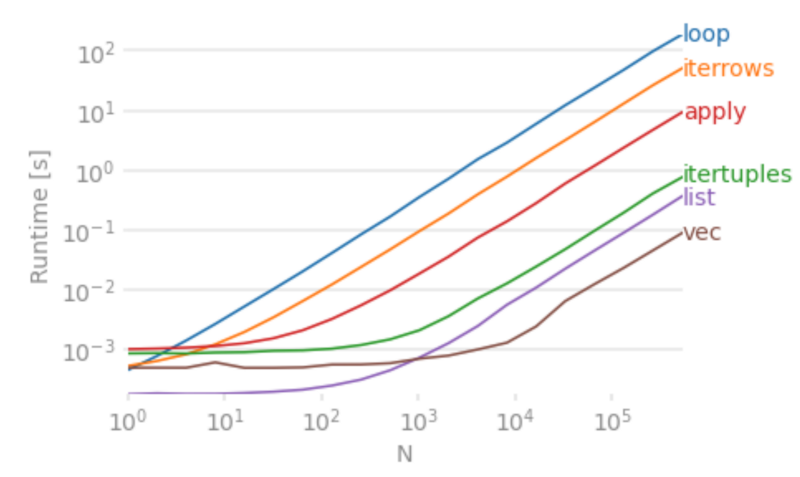# 6 Ways to Apply a Function to Every Row in Pandas DataFrameApplying a function to all rows in a Pandas DataFrame is one of the most common operations during data wrangling. There are many ways of doing it. In this article, you will measure the performance of 6 common alternatives. With a companion Colab, you can do it all in your browser. No need to install anything on your machine.

## Problem

Recently, I was analyzing user behavior data for an e-commerce app. Depending on the number of times a user did text and voice searches, I assigned each user to one of four cohorts:

• No Search: Users who did no search at all
• Text Only: Users who did text searches only
• Voice Only: Users who did voice searches only
• Both: Users who did both text and voice search

It was a huge data set with 100k to a million users depending upon the chosen time slice. Computing it with Pandas `apply()` function was excruciatingly slow, so I evaluated alternatives. This article is the distilled lessons from that.

I can’t share that dataset. So I am picking another similar problem to show the solutions: the Eisenhower method.Based on a task’s importance and urgency, the Eisenhower Method assigns it into one of 4 bins. Each bin has an associated action:

• Important and Urgent: Do right away
• Important but not Urgent: Schedule for later
• Not Important but Urgent: Delegate to someone else
• Neither Important nor Urgent: Delete time wasters.

We will use the boolean matrix shown in the adjacent figure. Importance and urgency booleans make the binary integer value for each action: DO(3), SCHEDULE(2), DELEGATE(1), DELETE(0).

We will profile the performance of mapping tasks to one of the actions. We will measure which of the 6 alternatives take the least amount of time. And we will plot the performance for up to a million tasks.

It is a good time to open the companion Colab. If you want to see the code in action, you can execute the cells in the Colab as you read along. Go ahead, execute all the cells in the Setup section.

## Test Data

Faker is a handy library to generate data. In the Colab, it is used for generating a DataFrame with a million tasks. Each task is a row in the DataFrame. It consists of task_name (`str`), due_date (`datetime.date`), and priority (`str`). Priority can be one of the three values: LOW, MEDIUM, HIGH.### Optimize DataFrame Storage

We will minimize the storage size to eliminate its effect on any of the alternatives. The DataFrame with a million tasks is taking 22.9MB:

``````>>> test_data_set.info()

<class 'pandas.core.frame.DataFrame'>
RangeIndex: 1000000 entries, 0 to 999999
Data columns (total 3 columns):
#   Column     Non-Null Count    Dtype
---  ------     --------------    -----
1   due_date   1000000 non-null  object
2   priority   1000000 non-null  object
dtypes: object(3)
memory usage: 22.9+ MB
``````

Instead of `str`, priority can be stored as Pandas `categorical` type:

``````priority_dtype = pd.api.types.CategoricalDtype(
categories=['LOW', 'MEDIUM', 'HIGH'],
ordered=True
)

test_data_set['priority'] = test_data_set['priority'].astype(priority_dtype)
``````

Let’s check out the DataFrame size now:

``````>>> test_data_set.info()

<class 'pandas.core.frame.DataFrame'>
RangeIndex: 1000000 entries, 0 to 999999
Data columns (total 3 columns):
#   Column     Non-Null Count    Dtype
---  ------     --------------    -----
1   due_date   1000000 non-null  object
2   priority   1000000 non-null  category
dtypes: category(1), object(2)
memory usage: 16.2+ MB
``````

Size is reduced to 16.2MB.

### Eisenhower Action Function

Given importance and urgency, `eisenhower_action` computes an integer value between 0 and 3.

``````def eisenhower_action(is_important: bool, is_urgent: bool) -> int:
return 2 * is_important + is_urgent
``````

For this exercise, we will assume that a task with HIGH priority is important. If the due date is in the next two days, then the task is urgent.

The Eisenhower Action for a task (i.e. a row in the DataFrame) is computed by using the `due_date` and `priority` columns:

``````>>> cutoff_date = datetime.date.today() + datetime.timedelta(days=2)

>>> eisenhower_action(
test_data_set.loc.priority == 'HIGH',
test_data_set.loc.due_date <= cutoff_date
)

2
``````

The integer 2 means that the needed action is to SCHEDULE.

In the rest of the article, we will evaluate 6 alternatives for applying `eisenhower_action` function to DataFrame rows. First, we will measure the time for a sample of 100k rows. Then, we will measure and plot the time for up to a million rows.

## Method 1: Loop Over Rows of Pandas DataFrame

The simplest method to process each row in the good old Python loop. This is obviously the worst way, and nobody in the right mind will ever do it.

``````def loop_impl(df):
cutoff_date = datetime.date.today() + datetime.timedelta(days=2)

result = []
for i in range(len(df)):
row = df.iloc[i]
result.append(
eisenhower_action(
row.priority == 'HIGH', row.due_date <= cutoff_date)
)

return pd.Series(result)
``````

As expected, it takes a horrendous amount of time: 37.5 seconds.

``````%timeit data_sample['action_loop'] = loop_impl(data_sample)

1 loop, best of 3: 37.5 s per loop
``````

It establishes the worst-case performance upper bound. Since its cost is linear, i.e. O(n), it provides a good baseline to compare other alternatives.

### Line Level Profiling

Let’s find out what is taking so long using the line_profiler, but for a smaller sample of 100 rows:

``````%lprun -f loop_impl  loop_impl(test_data_sample(100))
``````

Its output is shown in the following figure:Extracting a row from DataFrame (line #6) takes 90% of the time. Even if we take out that 90% cost from 37.5s for 100k rows, it would take 3.7s. That is still a lot of time.

## Method 2: Pandas DataFrame `iterrows` Function

Instead of processing each row in a Python loop, let’s try Pandas `[iterrows`](https://pandas.pydata.org/pandas-docs/stable/reference/api/pandas.DataFrame.iterrows.html) function.

``````def iterrows_impl(df):
cutoff_date = datetime.date.today() + datetime.timedelta(days=2)

return pd.Series(
eisenhower_action(
row.priority == 'HIGH', row.due_date <= cutoff_date)
for index, row in df.iterrows()
)
``````

It takes 9.59 seconds, approx. one-fourth of the time taken by the loop:

``````%timeit data_sample['action_iterrow'] = iterrows_impl(data_sample)

1 loop, best of 3: 9.59 s per loop
``````

## Method 3: Pandas DataFrame `itertuples` Function

Pandas has another method, `[itertuples`](https://pandas.pydata.org/pandas-docs/stable/reference/api/pandas.DataFrame.itertuples.html), that processes rows as tuples.

``````def itertuples_impl(df):
cutoff_date = datetime.date.today() + datetime.timedelta(days=2)

return pd.Series(
eisenhower_action(
row.priority == 'HIGH', row.due_date <= cutoff_date)
for row in df.itertuples()
)
``````

Its performance threw a surprise, it took only 178 milliseconds.

``````%timeit data_sample['action_itertuples'] = itertuples_impl(data_sample)

10 loops, best of 3: 178 ms per loop
``````

## Method 4: Pandas DataFrame `apply` Function

Pandas `[apply`](https://pandas.pydata.org/pandas-docs/stable/reference/api/pandas.DataFrame.apply.html) function is quite versatile and is a popular choice. To make it process the rows, you have to pass `axis=1` argument.

``````def apply_impl(df):
cutoff_date = datetime.date.today() + datetime.timedelta(days=2)
return df.apply(
lambda row:
eisenhower_action(
row.priority == 'HIGH', row.due_date <= cutoff_date),
axis=1
)
``````

This also threw a surprise for me. It took 1.75 seconds. 10x worse than `itertuples`!

``````%timeit data_sample['action_impl'] = apply_impl(data_sample)

1 loop, best of 3: 1.75 s per loop
``````

## Method 5: Python List Comprehension

A column in DataFrame is a Series that can be used as a list in a list comprehension expression:

``````[ foo(x) for x in df['x'] ]
``````

If multiple columns are needed, then `zip` can be used to make a list of tuples.

``````def list_impl(df):
cutoff_date = datetime.date.today() + datetime.timedelta(days=2)
return pd.Series([
eisenhower_action(priority == 'HIGH', due_date <= cutoff_date)
for (priority, due_date) in zip(df['priority'], df['due_date'])
])
``````

This also threw a surprise. It took only 70.7 milliseconds, even better than `itertuples`!

``````%timeit data_sample['action_list'] = list_impl(data_sample)

10 loops, best of 3: 70.7 ms per loop
``````

## Method 6: Vectorization

The real power of Pandas shows up in vectorization. But it requires unpacking the function as a vector expression using Pandas and NumPy.

``````def vec_impl(df):
cutoff_date = datetime.date.today() + datetime.timedelta(days=2)
return (
2*(df['priority'] == 'HIGH') + (df['due_date'] <= cutoff_date))
``````

It gives the best performance: only 19.5 milliseconds.

``````%timeit data_sample['action_vec'] = vec_impl(data_sample)

100 loops, best of 3: 19.5 ms per loop
``````

Vectorizing, depending upon the complexity of the function, can take significant efforts. Sometimes, it may not even be feasible.

## Plot Performance Over DataFrame Size

Plotting is helpful in understanding the relative performance of alternatives over input size. Perfplot is a handy tool for that. It requires a setup to generate input of a given size and a list of implementations to compare.

``````kernels = [
loop_impl,
iterrows_impl,
itertuples_impl,
apply_impl,
list_impl,
vec_impl
]

labels = [str(k.__name__)[:-5] for k in kernels]

perfplot.show(
setup=lambda n: test_data_sample(n),
kernels=kernels,
labels=labels,
n_range=[2**k for k in range(20)],
xlabel='N',
logx=True,
logy=True
)
``````

It generates a plot like the one shown below.Here are some observations from the plot:

• For this use case, asymptotic performance order stabilizes at about 10k rows in the DataFrame.
• List Comprehension is the best for less than a thousand rows.
• Since all lines in the plot become parallel, the perf difference might not be apparent in the log-log scale plot.
• The `itertuples` is as simple to use as `apply` but with 10x better performance
• List Comprehension is ~2.5x better than `itertuples`, though it can be verbose to write for a complex function
• Vectorization is ~3.5x and ~9x better than List Comprehension and `itertuples` respectively.

## Recommendations

Performing an operation independently to all Pandas rows is a common need. Here are my recommendations:

1. Pandas `itertuples` function: Its API is like `apply` function, but offers 10x better performance. It is the easiest and most readable option. It offers reasonable performance. It is now my default choice to start.
2. List Comprehension: When needing only 2–3 DataFrame columns, I opt for this alternative to squeeze better performance.
3. Vectorize DataFrame expression: I go for this whenever vectorization is straight forward. For performance-critical code, I try my best to vectorize and extract the best performance possible.

Understanding the cost of various alternatives is critical for making an informed choice. Use `timeit`, `line_profiler`, and `perfplot` to measure the performance of these alternatives. Balance performance with ease of use for deciding the best alternative for your use case.